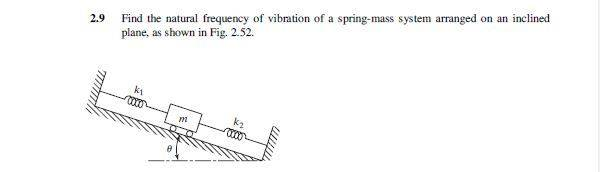# Natural frequency of a mass spring system

• whitejac

## Homework StatementT = 2π√(m/k)
mx'' + kx = 0
F = ma

## The Attempt at a Solution

I don't honestly know where to start with this. I'm really out of my league in this class, and I'm trying my best to catch up with the things I didn't quite master in Dynamics and Physics...
As far as I can tell, gravity pushes the mass down at a rate of:
m(9.8)(sinθ)

This then compresses k2 and elongates k1. So I should combine them into getting a keq because they are combined and he displacement is the same for each due to the mass moving linearly?

keq = (1/k1 + 1/k2)-1

That doesn't seem quite like i have enough because gravity is at play. If this were perfectly horizontal, there'd be nothing left but to plug keq back into the equation for T.

So I need to derive the relationship between gravity and the k coefficient because T doesn't imply any need for gravity to affect it, but it should. That's where I think I'm lost, though I probably went off track way earlier...

Take a look at the natural frequency equation for a simple mass-spring oscillator. Does it involve g in any way?

It doesn't, but wouldn't the consistent acceleration of gravity affect the frequency with which it would oscillate? If this were level, normal to gravity, and we neglected friction, a movement would oscillate it left and right. However, since it is tilted, it would also oscillate with the added pressing of its weight. I know the frequency formula shows no forced, but that just seems a bit too simple

With the cart on a slope as shown you can break the gravitational force into two components, one parallel to the slope and one perpendicular (normal). The normal component does not affect the motion of the system. The component parallel to the slope will produce a displacement 'bias', essentially a new equilibrium position for the cart. This is entirely equivalent to the case where a spring is stretched by a hanging mass so that the equilibrium point is below the natural length of the spring.

So... if I'm understanding this correctly then, it's plainly:
ω = √(keq / m)
Where keq = (1/k1 + 1/k2)-1

The reason we don't need to know any of the forces acting on it is because we already know the necessary components for ω = √(k/m).
If we had other components (like the length of the ramp and the position of the mass) then we would have to derive back towards that equation, but in this instance we do not have to because it was given.

Yup.

You might want to take a look at the short derivation on this page: Mass on Spring Resonance . The component of the gravitational force that parallels the slope in your problem is the analog of the mg term there.

That doesn't seem quite like i have enough because gravity is at play.
Check your intuition: does gravity matter?
Use a simpler situation with one mass and one spring... you know the answer when the spring is vertical right?